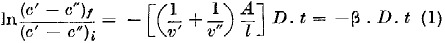# Equations for determining Diffusion Coefficients in Liquid Systems by the Diaphragm Cell Technique

## Abstract

THE diaphragm-cell technique, first introduced by Northrop and Anson1, has been widely used as an experimental method of utilizing Fick's first law to obtain diffusion coefficients in liquids. The procedure is a simple one whereby diffusion takes place through a porous diaphragm separating two cells in which the respective liquid concentrations, initially different to provide a driving force or potential for diffusion, are maintained uniform. It is made clear by Gordon2 in his classical review of the experimental and theoretical basis of the method that certain simplifying assumptions must be made in its utilization. These assumptions include constant solution volumes and constant fluxes throughout the porous diaphragm at any given moment. In measurements involving dilute solutions of electrolytes, the errors introduced by these assumptions have been analysed2,3 and found to be unimportant. In the present work the magnitude of the error introduced by the second assumption was assessed for typical diaphragm diffusion cell conditions (including consideration of a binary non-electrolyte system). Dropping this assumption, the resulting system of differential equations was solved by a numerical method. Comparison of the times required to produce a given concentration change with and without the above assumption showed a difference of not more than 0.02 per cent. The first assumption of constant solution volumes, however, may introduce significant error whenever the system is one that displays volume change on mixing. The importance of accurate liquid diffusion coefficients has led us to replace, with a more general expression, the simple logarithmic equation, given by Gordon2, that applies admittedly only if there is no change in volume on mixing :where c′ and c″ are concentrations, and v′ and v″ are volumes of the half-cells, ƒ and i refer to final and initial states respectively, A and l are the effective path area and length respectively, and β is the ‘cell factor’.

## Access options

from\$8.99

All prices are NET prices.

## References

1. 1

Northrop, J. H., and Anson, M. L., J. Gen. Physiol., 12, 543 (1929).

2. 2

Gordon, A. R., Ann. N.Y. Acad. Sci., 46, 285 (1945).

3. 3

Barnes, C., Physics, 5, 4 (1934).

4. 4

De Groot, S. R., “Thermodynamics of Irreversible Processes” (Interscience Pub., New York, 1951).

5. 5

Bird, R. B., in “Advances in Chemical Engineering”, 1 (Academic Press, New York, 1956).

## Rights and permissions

Reprints and Permissions

DULLIEN, F., SHEMILT, L. Equations for determining Diffusion Coefficients in Liquid Systems by the Diaphragm Cell Technique. Nature 187, 767–768 (1960) doi:10.1038/187767a0

• ### Diffusion coefficients for the liquid system: Ethanol-water

• F. A. L. Dullien
•  & L. W. Shemilt

The Canadian Journal of Chemical Engineering (1961)

• ### Activity-based Diffusion Coefficients for Liquid Solutions

• F. A. L. DULLIEN
•  & L. W. SHEMILT

Nature (1961)